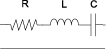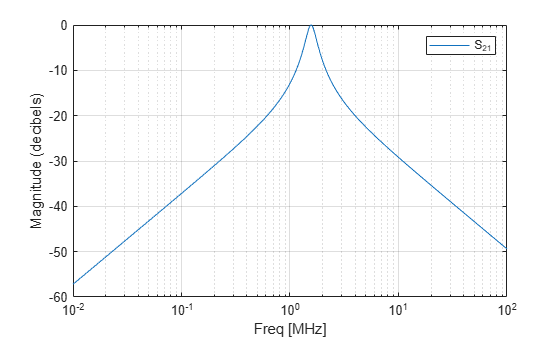# rfckt.seriesrlc

Series RLC component

## Description

Use the `rfckt.seriesrlc` object to create a two-port series RLC component as shown in this figure.Note

`seriesRLC` is recommended over `rfckt.seriesrlc` because it enables you to:

(since R2023b)

## Creation

### Syntax

``h = rfckt.seriesrlc``
``h = rfckt.seriesrlc('R',Rvalue,'L',Lvalue,'C',Cvalue)``

### Description

example

````h = rfckt.seriesrlc` returns a series RLC network object whose properties all have their default values. The default object is equivalent to a pass-through 2-port network, i.e., the resistor, inductor, and capacitor are each replaced by a short circuit.```
````h = rfckt.seriesrlc('R',Rvalue,'L',Lvalue,'C',Cvalue)` sets properties using one or more name-value pairs. You can specify multiple name-value pairs. Enclose each property name in a quote```

## Properties

expand all

Computed S-parameters, noise figure, OIP3, and group delay values, specified as rfdata.data object. `Analyzed Result` is a read-only property. For more information refer, Algorithms.

Data Types: `function_handle`

Resistance value, specified as a positive scalar in ohms. The default value is `0`.

Data Types: `double`

Capacitance value, specified as a positive scalar in farads. The default value is `'Inf'`.

Data Types: `double`

Inductance value, specified as a positive scalar in henries. The default value is `0`.

Data Types: `double`

Object name, specified as a `1-by-N` character array. `Name` is a read-only property.

Data Types: `char`

Number of ports, specified as a positive integer. `nport`t is a read-only property. The default value is `2`.

Data Types: `double`

## Object Functions

 `analyze` Analyze RFCKT object in frequency domain `calculate` Calculate specified parameters for rfckt objects or rfdata objects `circle` Draw circles on Smith Chart `extract` Extract specified network parameters from rfckt object or data object `listformat` List valid formats for specified circuit object parameter `listparam` List valid parameters for specified circuit object `loglog` Plot specified circuit object parameters using log-log scale `plot` Plot circuit object parameters on X-Y plane `plotyy` Plot parameters of RF circuit or RF data on X-Y plane with two Y-axes `getop` Display operating conditions `polar` Plot specified object parameters on polar coordinates `semilogx` Plot RF circuit object parameters using log scale for x-axis `semilogy` Plot RF circuit object parameters using log scale for y-axis `smith` Plot circuit object parameters on Smith chart `write` Write RF data from circuit or data object to file `getz0` Calculate characteristic impedance of RFCKT transmission line object `read` Read RF data from file to new or existing circuit or data object `restore` Restore data to original frequencies `getop` Display operating conditions `groupdelay` Group delay of S-parameter object or RF filter object or RF Toolbox circuit object

## Examples

collapse all

This example creates a series LC resonator and examines its frequency response. It first creates the circuit object and then uses the analyze method to calculate its frequency response. Finally, it plots the results - first, the magnitude in decibels (dB):

```h = rfckt.seriesrlc('L',4.7e-5,'C',2.2e-10); analyze(h,logspace(4,8,1000)); plot(h,'s21','dB') ax = gca; ax.XScale = 'log';```The example then plots the phase, in degrees:

```figure plot(h,'s21','angle') ax = gca; ax.XScale = 'log';```## Algorithms

The `analyze` method computes the S-parameters of the `AnalyzedResult` property using the data stored in the `rfckt.seriesrlc` object properties by first calculating the ABCD-parameters for the circuit, and then converting the ABCD-parameters to S-parameters using the `abcd2s` function. For this circuit, A = 1, B = Z, C = 0, and D = 1, where

`$Z=\frac{-LC{\omega }^{2}+jRC\omega +1}{jC\omega }$`

and ω = 2πf.

The `analyze` method uses the S-parameters to calculate the group delay values at the frequencies specified in the `analyze` input argument `freq`, as described in the `analyze` reference page.

 Ludwig, Reinhold and Pavel Bretchko, RF Circuit Design: Theory and Applications, Prentice-Hall, 2000.